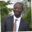Related Tags

javascript
communitycreator
array

# How to sum a JavaScript array with reduceAbel Lifaefi Mbula

In the previous shot, we learned how to get the sum of an array using three different methods. Today, we want to learn how to do so using the reduce method.

With all the three methods we’ve learned, we’ve noticed one common thing: code mutation.

Let’s see an example.

function sumArray(array) {
let sum = 0;

for (let i = 0; i < array.length; i += 1) {
sum += array[i];
}

return sum;
}

console.log(sumArray([1, 4, 0, 9, -3])); // sum to 11


As you can see here, we first declare a variable (sum) and set its initial value to 0. Then, thanks to the for loop, we iterate through the array and add each array item to sum  to get the total.

While this traditional way looks pretty simple, there’s a real issue we must be aware of: code mutation. We first set an initial value for sum, and modify it later. Code mutation is bad because it makes our code more error-prone and less readable. We need to avoid this as much as we can.

### The modern way

The code above is an example of a code mutation. To avoid this, JavaScript provides us with a built-in solution: reduce(). Let’s see how to use it.

reduce accepts two mandatory parameters: the accumulator (total) and the currentValue (item). reduce cycles through each number in the array, much like it would in a traditional for loop.

function sumArray(array) {
/*Go through array and loop
total starting point is 1 and item = 4
*/
const sum = array.reduce((total, item) => total + item);

/*
1st call: total = 1, item = 4 => sum = 1 + 4 = 5
2nd call: total = 5, item = 0 => sum = 5 + 0 = 5
3rd call: total = 5, item = 9 => sum = 5 + 9 = 14
4th call: total = 14, item = -3 => sum = 14 + -3 = 11
*/

// return the result
console.log(sum);
return sum;
}

sumArray([1, 4, 0, 9, -3]); //logs 11


Notice that we made four calls instead of five. That is because reduce initially takes the first and second items, so next time we jump to the third item. When there are no more numbers left in the array, the method returns the total value.

If you are afraid of arrow functions, here is the traditional way:

function sumArray(array) {
/*Go through array and loop
total starting point is 1 and item = 4
*/
const sum = array.reduce(function(total, item) {
});

/*
1st call: total = 1, item = 4 => sum = 1 + 4 = 5
2nd call: total = 5, item = 0 => sum = 5 + 0 = 5
3rd call: total = 5, item = 9 => sum = 5 + 9 = 14
4th call: total = 14, item = -3 => sum = 14 + -3 = 11
*/

// return the result
console.log(sum);
return sum;
}

sumArray([1, 4, 0, 9, -3]); //logs 11


function sumArray(array) {

const sum = array.reduce((total, item) => total + item);

console.log(sum);
return sum;
}

sumArray([1, 4, 0, 9, -3]);


### Old-fashioned

function sumArray(array) {

const sum = array.reduce(function(total, item) {
});

console.log(sum);
return sum;
}

sumArray([1, 4, 0, 9, -3]);


### That’s a wrap

We’ve learned that code mutation is bad and we should avoid it as much as we can. reduce() is a built-in method that helps us avoid code mutation, as we can see it using the for loop.

RELATED TAGS

javascript
communitycreator
array

CONTRIBUTORAbel Lifaefi Mbula
RELATED COURSES

View all Courses

Keep Exploring

Learn in-demand tech skills in half the time